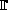# Polynomial order

Let P(x) be an irreducible polynomial of degree d>1 over a prime finite fieldp. The order of P is the smallest positive integer n such that P(x) divides xn-1. n is also equal to the multiplicative order of any root of P. It is a divisor of pd-1. P is a primitive polynomial if n=pd-1.

This tool allows you to enter a polynomial and compute its order. If you enter a reducible polynomial, the orders of all its non-linear factors will be computed and presented.

Enter your polynomial: ( Help: how to enter a polynomial )

Over the finite fieldp of characteristics p = .

In order to access WIMS services, you need a browser supporting forms. In order to test the browser you are using, please type the word wims here: and press Enter''.